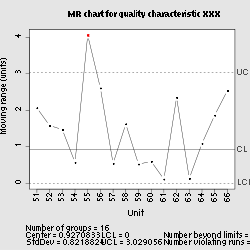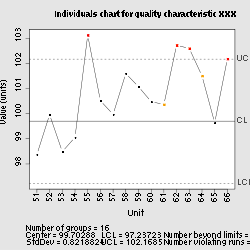# Shewhart individuals control chart

﻿
Shewhart individuals control chart
Individuals and moving range control chart
Originally proposed by Walter A. Shewhart
Process observations
Rational subgroup size n = 1
Measurement type Average quality characteristic per unit
Quality characteristic type Variables data
Underlying distribution Normal distribution
Performance
Size of shift to detect ≥ 1.5σ
Process variation chartCenter line$\overline{MR_i} = \frac {\sum_{i=2}^m \big| x_i - x_{i - 1} \big| }{m - 1}$
Upper control limit$D_4 \overline{MR}$
Lower control limit$D_3 \overline{MR}$
Plotted statistic$MR_i = \big | x_i - x_{i - 1} \big |$
Process mean chartCenter line$\overline{x} = \frac {\sum_{i=1}^m x_{i}}{m}$
Control limits$\overline{x} \pm 3\frac {\overline{MR}}{d_2}$
Plotted statistic xi

In statistical quality control, the individual/moving-range chart is a type of control chart used to monitor variables data from a business or industrial process for which it is impractical to use rational subgroups.

The chart is necessary in the following situations::231

1. Where automation allows inspection of each unit, so rational subgrouping has no benefit.
2. Where production is slow so that waiting for enough samples to make a rational subgroup unacceptably delays monitoring
3. For processes that produce homogeneous batches (e.g., chemical) where repeat measurements vary primarily because of measurement error

The "chart" actually consists of a pair of charts: one, the individuals chart, displays the individual measured values; the other, the moving range chart, displays the difference from one point to the next. As with other control charts, these two charts enable the user to monitor a process for shifts in the process that alter the mean or variance of the measured statistic.

## Interpretation

As with other control charts, the individuals and moving range charts consist of points plotted with the control limits, or natural process limits. These limits are the voice of the process, and define what the process will deliver without fundamental changes to the process.:43 Points outside of these control limits are signals indicating that the process is not operating as consistently as possible; that some assignable cause has resulted in a change in the process. Similarly, runs of points on one side of the average line should also be interpreted as a signal of some change in the process. When such signals exist, action should be taken to identify and eliminate them. When no such signals are present, no changes to the process control variables (i.e. "tampering") are necessary or desirable.:125

## Assumptions

The normal distribution is assumed in the calculation of control limits. As with other control charts, deviations from the following criteria will increase the likelihood of falsely detecting signals:

• The quality characteristic to be monitored is adequately modeled by a normally-distributed random variable;
• The parameters μ and σ for the random variable are the same for each unit and each unit is independent of its predecessors or successors;
• The inspection procedure is same for each sample and is carried out consistently from sample to sample.

However, because of the width of the control limits and the use of the charts to diagnose the existence of special causes, individuals charts are reasonably robust to deviations from the above assumptions. This is demonstrated by Wheeler using real-world data,  and for a number of highly non-normal probability distributions.

## Calculation and plotting

### Calculation of moving range

The difference between data point, xi, and its predecessor, xi − 1, is calculated as${MR}_i = \big| x_i - x_{i - 1} \big|$. For m individual values, there are m − 1 ranges.

Next, the arithmetic mean of these values is calculated as$\overline{MR}=\frac{\sum_{i=2}^{m}{MR_i}}{m-1}$

If the data are normally distributed with standard deviation σ then the expected value of$\overline{MR}$ is$d_{2} \sigma= 2\sigma/\sqrt \pi .$

### Calculation of moving range control limit

The upper control limit for the range (or upper range limit) is calculated by multiplying the average of the moving range by 3.267:$UCL_r = 3.267\overline{MR}$.

The value 3.267 is taken from the sample size-specific D4 anti-biasing constant for n=2, as given in most textbooks on statistical process control (see, for example, Montgomery:725).

### Calculation of individuals control limits

First, the average of the individual values is calculated:$\overline{x}=\frac{\sum_{i=1}^{m}{x_i}}{m}$.

Next, the upper control limit (UCL) and lower control limit (LCL) for the individual values (or upper and lower natural process limits) are calculated by adding or subtracting 2.66 times the average moving range to the process average:$UCL=\overline{x}+2.66\overline{MR}$$LCL=\overline{x}-2.66\overline{MR}$

The value 2.66 is obtained by dividing 3 by the sample size-specific d2 anti-biasing constant for n=2, as given in most textbooks on statistical process control (see, for example, Montgomery:725).

### Creation of graphs

Once the averages and limits are calculated, all of the individuals data are plotted serially, in the order in which they were recorded. To this plot is added a line at the average value, x and lines at the UCL and LCL values.

On a separate graph, the calculated ranges MRi are plotted. A line is added for the average value, MR and second line is plotted for the range upper control limit (UCLr).

### Analysis

The resulting plots are analyzed as for other control charts, using the rules that are deemed appropriate for the process and the desired level of control. At the least, any points above either upper control limits or below the lower control limit are marked and considered a signal of changes in the underlying process that are worth further investigation.

## Potential pitfalls

The moving ranges involved are serially correlated so runs or cycles can show up on the moving average chart that do not indicate real problems in the underlying process.:237

In some cases, it may be advisable to use the median of the moving range rather than its average, as when the calculated range data contains a few large values that may inflate the estimate of the population's dispersion.

Departures in normality in the process output significantly reduce the effectiveness of the charts to the point where it may require control limits to be set based on percentiles of the empirically-determined distribution of the process output.:237

Many software packages will, given the individuals data, perform all of the needed calculations and plot the results. Care should be taken to ensure that the control limits are correctly calculated, per the above and standard texts on SPC. In some cases, the software's default settings may produce incorrect results; in others, user modifications to the settings could result in incorrect results. Sample data and results are presented by Wheeler for the explicit purpose of testing SPC software. Performing such software validation is generally a good idea with any SPC software.

Wikimedia Foundation. 2010.

### Look at other dictionaries:

• Control chart — One of the Seven Basic Tools of Quality First described by Walter A. Shewhart …   Wikipedia

• Multi-vari chart — In quality control, multi vari charts are a visual way of presenting variability through a series of charts. The content and format of the charts has evolved over time. Original concept Multi vari charts were first described by Leonard Seder in… …   Wikipedia

• p-chart — Originally proposed by Walter A. Shewhart Process observations Rational subgroup size n > 1 Measurement type Fraction nonconforming in a sample Quality characteristic type Attributes d …   Wikipedia

• контрольная карта — 3.1 контрольная карта (control chart): График, на который наносят в установленном порядке значения статистического показателя в последовательности выборок, используемый для управления процессом и снижения изменчивости процесса. Примечание 1… …   Словарь-справочник терминов нормативно-технической документации

• ГОСТ Р ИСО 7870-1-2011: Статистические методы. Контрольные карты. Часть 1. Общие принципы — Терминология ГОСТ Р ИСО 7870 1 2011: Статистические методы. Контрольные карты. Часть 1. Общие принципы оригинал документа: 3.28 автокорреляции (autocorrelation): Корреляция между наблюдениями характеристики, упорядоченными по времени. [ИСО 3534 2 …   Словарь-справочник терминов нормативно-технической документации

• Six Sigma — Not to be confused with Sigma 6. The often used Six Sigma symbol Part of a series of articles on I …   Wikipedia

• Quality assurance — Quality assurance, or QA for short, refers to planned and systematic production processes that provide confidence in a product s suitability for its intended purpose. It is a set of activities intended to ensure that products (goods and/or… …   Wikipedia